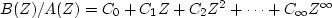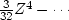Next: INTRODUCTION TO ALL-PASS FILTERS Up: MINIMUM-PHASE FILTERS Previous: Mechanical interpretation

## Laurent expansion

Given an unknown filter B(Z), to understand its inverse, we need to factor B(Z) into two parts: B(Z) = Bout(Z) Bin(Z), where Bout contains all the roots outside the unit circle and Bin contains all the roots inside. Then the inverse of Bout is expressed as a Taylor series about the origin, and the inverse of Bin is expressed as a Taylor series about infinity. The final expression for 1/B(Z) is called a Laurent expansion'' for 1/B(Z), and it converges on a ring including the unit circle. Cases with zeros exactly on the unit circle present special problems. For example, the differentiation filter (1-Z) is the inverse of integration, but the converse is not true, because of the additive constant of integration.

## EXERCISES:

1. Find the filter that is inverse to (2 - 5Z + 2Z2). You may just drop higher-order powers of Z, but an exact expression for the coefficients of any power of Z is preferable. (Partial fractions is a useful, though not a necessary, technique.) Sketch the impulse response.
2. Describe a general method for determining A(Z) and B(Z) from a Taylor series of,where B(Z) and A(Z) are polynomials of unknown degree n and m, respectively. Work out the case.Do not try this problem unless you are familiar with determinants. (HINT: identify coefficients of B(Z) = A(Z) C(Z).)Next: INTRODUCTION TO ALL-PASS FILTERS Up: MINIMUM-PHASE FILTERS Previous: Mechanical interpretation
Stanford Exploration Project
10/21/1998